# [Crypto] gmpy2教程大全

2主题 0精华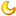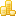学币
5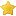荣耀
0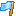rank
0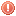违规
0发表于 2021-7-7 17:35:29 | 显示全部楼层 |阅读模式1.如果您喜欢这篇帖子，请给作者点赞评分，点赞会增加帖子的热度，评分会给作者加学币。(评分不会扣掉您的积分，系统每天都会重置您的评分额度)。
2.回复帖子不仅是对作者的最好奖励，还可以获得学币奖励，请尊重作者的劳动成果，拒绝做伸手党！
3.发广告、灌水回复等违规行为一经发现直接禁言，如果本帖内容涉嫌违规，请点击论坛底部的举报反馈按钮，也可以在【投诉建议】板块发帖举报。

### 相关帖子

2主题 0精华学币
5荣耀
0rank
0违规
0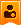楼主| 发表于 2021-7-7 17:36:04 | 显示全部楼层
 本帖最后由 ctfer 于 2021-7-7 17:41 编辑 该MPZ类型与Python的内置INT /长型兼容，但significanly更快较大的值。性能的转换点各不相同，但可以低至 20 到 40 位数字。提供了多种附加的整数函数。>>>importr gmpy2 >>>form gmpy2 import mpz,mpq,mpfr,mpc >>> mpz ( 99 )  *  43 mpz(4257) >>> pow ( mpz ( 99 ),  37 ,  59 ) mpz(18) >>> gmpy2.isqrt ( 99 ) mpz(9) >>> gmpy2.isqrt_rem ( 99 ) (mpz(9), mpz(18)) >>> gmpy2.gcd ( 123 , 27 ) mpz(3) >>> gmpy2.lcm ( 123 , 27 ) mpz(1107) 该MPQ类型与兼容fractions.Fraction包括在Python类型。>>> mpq ( 3 , 7 ) / 7 mpq(3,49) >>> mpq ( 45 , 3 )  *  mpq ( 11 , 8 ) mpq(165,8) gmpy2 中最重要的新特性是支持基于 MPFR 和 MPC 库的正确舍入的任意精度实数和复数算术。浮点上下文用于控制异常情况。例如，除以零可以返回 Infinity 或引发异常。>>> mpfr(1)/7 mpfr('0.14285714285714285') >>> gmpy2.get_context().precision=200 >>> mpfr(1)/7 mpfr('0.1428571428571428571428571428571428571428571428571428571428571',200) >>> gmpy2.get_context() context(precision=200, real_prec=Default, imag_prec=Default,         round=RoundToNearest, real_round=Default, imag_round=Default,         emax=1073741823, emin=-1073741823,         subnormalize=False,         trap_underflow=False, underflow=False,         trap_overflow=False, overflow=False,         trap_inexact=False, inexact=True,         trap_invalid=False, invalid=False,         trap_erange=False, erange=False,         trap_divzero=False, divzero=False,         trap_expbound=False,         allow_complex=False) >>> mpfr(1)/0 mpfr('inf') >>> gmpy2.get_context().trap_divzero=True >>> mpfr(1)/0 Traceback (most recent call last):   File "", line 1, in gmpy2.DivisionByZeroError: 'mpfr' division by zero in division >>> gmpy2.get_context() context(precision=200, real_prec=Default, imag_prec=Default,         round=RoundToNearest, real_round=Default, imag_round=Default,         emax=1073741823, emin=-1073741823,         subnormalize=False,         trap_underflow=False, underflow=False,         trap_overflow=False, overflow=False,         trap_inexact=False, inexact=True,         trap_invalid=False, invalid=False,         trap_erange=False, erange=False,         trap_divzero=True, divzero=True,         trap_expbound=False,         allow_complex=False) >>> gmpy2.sqrt(mpfr(-2)) mpfr('nan') >>> gmpy2.get_context().allow_complex=True >>> gmpy2.get_context().precision=53 >>> gmpy2.sqrt(mpfr(-2)) mpc('0.0+1.4142135623730951j') >>> >>> gmpy2.set_context(gmpy2.context()) >>> with gmpy2.local_context() as ctx: ...   print(gmpy2.const_pi()) ...   ctx.precision+=20 ...   print(gmpy2.const_pi()) ...   ctx.precision+=20 ...   print(gmpy2.const_pi()) ... 3.1415926535897931 3.1415926535897932384628 3.1415926535897932384626433831 >>> print(gmpy2.const_pi()) 3.1415926535897931 >>> 杂项 gmpy2 函数from_binary(...) from_binary(bytes) 从 to_binary() 创建的字节序列返回一个 gmpy2 对象。 get_cache(...)get_cache() 返回当前缓存大小（对象数）和每个对象的最大大小（肢体数）。gmpy2 维护一个已释放的mpz、xmpz、mpq、mpfr和 mpc对象的内部列表以供重用。缓存显着提高了性能，但也增加了内存占用。 license（...） license() 返回 gmpy2 许可证信息。 mp_limbsize(...) mp_limbsize() 返回 GMP 或 MPIR 库使用的每个肢体的位数。 mp_version(...) mp_version() 返回 GMP 或 MPIR 库的版本。 mpc_version(...) mpc_version() 返回 MPC 库的版本。 mpfr_version(...) mpfr_version() 返回 MPFR 库的版本。 random_state（...） random_state([seed]) 返回一个包含随机数生成器状态信息的新对象。可以将可选的整数参数指定为种子值。仅支持 Mersenne Twister 随机数生成器。 set_cache(...)set_cache(number, size) 更新缓存的每种类型的最大释放对象数和每个对象的最大大小（以肢体为单位）。可以缓存的每种类型的最大对象数为 1000。一个对象的最大大小为 16384。一个对象的最大大小在 32 位系统上约为 64K，在 64 位系统上约为 128K。

2主题 0精华学币
5荣耀
0rank
0违规
0楼主| 发表于 2021-7-7 17:47:38 | 显示全部楼层
 本帖最后由 ctfer 于 2021-7-7 17:48 编辑 多精度整数gmpy2 mpz类型支持任意精度整数。它应该是 Python 的long类型的替代品。根据平台和具体操作的不同，一旦精度超过 20 到 50 位，mpz将比 Python 的long更快。支持 GMP 中的所有特殊整数函数。例子>>> import gmpy2 >>> from gmpy2 import mpz >>> mpz('123') + 1 mpz(124) >>> 10 - mpz(1) mpz(9) >>> gmpy2.is_prime(17) True mpz 方法 bit_clear(...) x.bit_clear(n) 返回x的副本，其中位n设置为 0。 x.bit_flip（...） x.bit_flip(n) 返回x的副本，其中位n反转。 x.bit_length(...) x.bit_length() 返回x的基数 2 表示中的有效位数。为了与 Python 兼容，mpz(0).bit_length() 返回 0。 bit_scan0(...) x.bit_scan0(n) 返回x的第一个 0 位的索引，索引 >= n。如果x 中在索引n处或以上没有更多的 0 位 （这只能在x < 0 时发生，假设无限长的 2 的补码格式），则返回 None 。n必须 >= 0。 bit_scan1(...) x.bit_scan1(n) 返回x的第一个 1 位的索引，索引 >= n。如果x 中在索引n处或以上没有更多的 1 位 （这只能在x >= 0 时发生，假设无限长 2 的补码格式），则返回 None 。n必须 >= 0。 x.bit_set（...） x.bit_set(n) 返回x的副本，其中位n设置为 1。 x.bit_test（...） 如果设置了x 的第n位，则 x.bit_test(n) 返回 True，如果未设置则返回False。 x.denominator(...) x.denominator() 返回 mpz(1)。 x.digits(...) x.digits([base=10]) 返回一个以基数base表示x的字符串。 x.numerator（...） x.numerator() 返回 x 的副本。 num_digits(...) x.num_digits（[碱= 10]）返回表示的绝对值的字符串的长度X在基数基。如果基数是 2 的幂，则结果正确。对于其他其他基数，结果通常是正确的，但可能 1 太大。base 的范围可以在 2 到 62 之间，包括 2 和 62。 mpz 函数 add（...） add(x, y) 返回x + y。结果类型取决于输入类型。 bincoef(...) bincoef(x, n) 返回二项式系数。n必须 >= 0。 bit_clear(...) bit_clear(x, n) 返回x的副本，其中位n设置为 0。 bit_flip（...） bit_flip(x, n) 返回x的副本，其中位n反转。 BIT_LENGTH(...) BIT_LENGTH（x）返回在的基2表示显著的比特数X。为了与 Python 兼容，mpz(0).bit_length() 返回 0，而 mpz(0).num_digits(2) 返回 1。 bit_mask（...） bit_mask(n) 返回一个mpz对象，它的长度正好是n位，所有位都已设置。 bit_scan0(...) bit_scan0（X，N）返回的第一个0比特的索引X与指数> = Ñ。如果x 中在索引n处或以上没有更多的 0 位 （这只能在x < 0 时发生，假设无限长的 2 的补码格式），则返回 None 。n必须 >= 0。 bit_scan1(...) bit_scan1（X，N）返回的第一1比特的索引X与指数> = Ñ。如果x 中在索引n处或以上没有更多的 1 位 （这只能在x >= 0 时发生，假设无限长 2 的补码格式），则返回 None 。n必须 >= 0。 bit_set（...） bit_set(x, n) 返回x的副本，其中位n设置为 1。 bit_test（...） 如果设置了x 的第n位，则 bit_test(x, n) 返回 True，如果未设置则返回False。 c_div(...) c_div(x, y) 返回x除以y的商。商向 +Inf（上限四舍五入）四舍五入。x和y必须是整数。 c_div_2exp(...) c_div_2exp(x, n) 返回x除以 2**n的商。商向 +Inf（上限四舍五入）四舍五入。x必须是整数，n必须 > 0。 c_divmod(...) c_divmod(x, y) 返回x除以 y的商和余数。商向 +Inf（上限四舍五入）四舍五入，余数将与y符号相反。x和y必须是整数。 c_divmod_2exp(...) c_divmod_2exp(x ,n) 返回x除以 2**n的商和余数。商向 +Inf（上限四舍五入）四舍五入，余数将为负数或零。x必须是整数，n必须 > 0。 c_mod(...) c_mod(x, y) 返回x除以y的余数。余数将具有与y相反的符号。x和y必须是整数。 c_mod_2exp(...) c_mod_2exp(x, n) 返回x除以 2**n的余数。余数将为负。x必须是整数，n必须 > 0。 comb（...） comb(x, n) 返回x事物的组合数，一次取n 。n必须 >= 0。 div(...) div(x, y) 返回x / y。结果类型取决于输入类型。 diverxact(...) diverxact(x, y) 返回x除以y的商。比标准除法快但要求余数为零！ div(...) divm(a, b, m) 返回x使得b * x == a modulo m。如果不存在这样的值x，则引发 ZeroDivisionError 异常。 f_div(...) f_div(x, y) 返回x除以y的商。商向 -Inf（地板四舍五入）四舍五入。x和y必须是整数。 f_div_2exp(...) f_div_2exp(x, n) 返回x除以 2**n的商。商向 -Inf（地板四舍五入）四舍五入。x必须是整数，n必须 > 0。 f_divmod(...) f_divmod(x, y) 返回x除以 y的商和余数。商向 -Inf（地板四舍五入）四舍五入，余数将与y具有相同的符号。x和y必须是整数。 f_divmod_2exp(...) f_divmod_2exp(x, n) 在将x 除以 2**n后返回商和余数。商向 -Inf（下舍入）四舍五入，余数将为正。x必须是整数，n必须 > 0。 f_mod(...) f_mod(x, y) 返回x除以y的余数。余数将与y具有相同的符号。x和y必须是整数。 f_mod_2exp(...) f_mod_2exp(x, n) 返回x除以 2**n 的余数。余数将是正数。x必须是整数，n必须 > 0。 FAC（...） FAC（n）返回的确切阶乘Ñ。使用 factorial() 获得浮点近似值。 fib（...） fib(n) 返回第n个斐波那契数。 fib2(...) fib2(n) 返回一个包含 ( n -1) 和n斐波那契数的 2 元组。 gcd(...) gcd(a, b) 返回整数a和 b 的最大公约数。 gcdext(...) gcdext(a, b) 返回一个 3 元素元组 ( g , s , t ) 使得g == gcd( a , b ) 和g == a * s + b * t hamdist(...) hamdist(x, y) 返回整数x和y之间的汉明距离（位不同的位位置数）。 invert（...） invert(x, m) 返回y使得x * y == 1 modulo m，如果不存在这样的y则返回0 。 iroot(...) iroot(x,n) 返回一个 2 元素元组 ( y , b )，其中y是x的整数 n 次根，如果根是精确的，则b为 True。x必须 >= 0，n必须 > 0。 iroot_rem(...) iroot_rem(x,n) 返回一个 2 元素元组 ( y , r )，其中y是x的整数n -th 根且x = y**n + r。x必须 >= 0， n必须 > 0。 is_even（...） 如果x是偶数，is_even(x) 返回 True ，否则返回False。 is_odd(...) 如果x是奇数，is_odd(x) 返回 True ，否则返回False。 is_power(...) 如果x是完美幂，则 is_power(x) 返回 True ，否则返回False。 is_prime(...) is_prime（X [中，n = 25]）如果返回True X是大概素数。如果x肯定是复合的，则返回 False 。检查x的小除数，并执行最多n 次Miller-Rabin 测试。实际执行的测试可能因所使用的 GMP 或 MPIR 版本而异。 is_square(...) 如果x是一个完美的平方，is_square(x) 返回 True ，否则返回False。 isqrt(...) isqrt（x）返回一个整数的整数平方根X。x必须 >= 0。 isqrt_rem(...) isqrt_rem(x) 返回一个二元组 ( s , t ) 使得s = isqrt( x ) 和t = x - s * s。x必须 >= 0。 jacobi(...) jacobi(x, y) 返回雅可比符号 ( x | y )。y必须是奇数且 > 0。 kronecker(...) kronecker(x, y) 返回 Kronecker-Jacobi 符号 ( x | y )。 lcm(...) lcm(a, b) 返回整数a和b的最小公倍数。 Legendre(...) Legendre(x, y) 返回 Legendre 符号 ( x | y )。y被假定为奇素数。 lucas(...) lucas(n) 返回第n个卢卡斯数。 lucas2(...) lucas2(n) 返回一个带有 ( n -1)-th 和n -th Lucas 数的 2 元组。 mpz(...) mpz() 返回设置为 0的新mpz对象。 mpz(n)从数值n返回一个新的mpz对象。如果n不是整数，它将被截断为整数。 mpz(s[, base=0])从由给定基数中的数字组成的字符串s 中返回一个新的mpz对象。如果 base = 0，则二进制、八进制或十六进制 Python 字符串由前导 0b、0o 或 0x 字符识别。否则字符串被假定为十进制。base 的值可以在 2 到 62 之间。 mpz_random(...) mpz_random(random_state, n) 返回一个介于 0 和n -1之间的均匀分布的随机整数。参数random_state必须首先由 random_state() 创建。 mpz_rrandomb(...) mpz_rrandomb(random_state, b) 返回一个介于 0 和 2**b - 1 之间的随机整数，其二进制表示形式为 0 和 1 的长序列。参数random_state必须首先由 random_state() 创建。 mpz_urandomb(...) mpz_urandomb(random_state, b) 返回一个介于 0 和 2**b - 1 之间的均匀分布的随机整数。 参数random_state必须首先由 random_state() 创建。 mul（...） mul(x, y) 返回x * y。结果类型取决于输入类型。 next_prime(...) next_prime(x) 返回下一个可能的素数 > x。 num_digits(...) NUM_DIGITS（X [，碱= 10]）返回表示的绝对值的字符串的长度X在基数基。如果基数是 2 的幂，则结果正确。对于其他其他基数，结果通常是正确的，但可能 1 太大。base 的范围可以在 2 到 62 之间，包括 2 和 62。 popcount(...) popcount（x）返回与值1的位的数目X。如果x < 0，则值为 1 的位数是无限的，因此在这种情况下返回 -1。 powmod(...) powmod(x, y, m) 返回 ( x ** y ) mod m。指数y可以是负数，如果x mod m的倒数存在，则将返回正确的结果。否则，会引发 ValueError。 remove（...） remove(x, f) 将尽可能多地从x 中删除因子f并返回一个 2 元组 ( y , m ) 其中y = x // ( f ** m )。f不除以y。m是x 中因子f的重数。f必须 > 1。 sub(...) sub(x, y) 返回x - y。结果类型取决于输入类型。 t_div(...) t_div(x, y) 返回x除以y的商。商向零四舍五入（截断）。x和y必须是整数。 t_div_2exp(...) t_div_2exp(x, n) 返回x除以 2**n的商。商向零四舍五入（截断）。n必须 > 0。 t_divmod(...) t_divmod(x, y) 返回x除以 y的商和余数。商向零舍入（截断），余数将与x具有相同的符号。x和y必须是整数。 t_divmod_2exp(...) t_divmod_2exp(x, n) 返回x除以 2**n的商和余数。商向零舍入（截断），余数将与x具有相同的符号。x必须是整数，n 必须 > 0。 t_mod(...) t_mod(x, y) 返回x除以y的余数。余数将与x具有相同的符号。x和y必须是整数。 t_mod_2exp(...) t_mod_2exp(x, n) 返回x除以 2**n的余数。余数将与x具有相同的符号。x必须是整数，n 必须 > 0。

2主题 0精华学币
5荣耀
0rank
0违规
0楼主| 发表于 2021-7-7 17:51:54 | 显示全部楼层
 多精度整数（高级主题）xmpz 类型gmpy2 提供对称为xmpz的实验性整数类型的访问。所述 xmpz类型是可变的整数类型。就地操作（+=、//= 等）修改原始对象并且不创建新对象。xmpz 的实例 不能用作字典键。>>> import gmpy2 >>> from gmpy2 import xmpz >>> a = xmpz(123) >>> b = a >>> a += 1 >>> a xmpz(124) >>> b xmpz(124)复制代码 就地更改xmpz对象的能力允许有效和快速的位操作。 可以设置或清除单个位：>>> a [ 10 ] = 1 >>> a xmpz(1148)复制代码支持切片符号。切片引用的位可以是“读取”或“写入”。要清除位片，请使用源值 0。在 2s 补码格式中，0 由任意数量的 0 位表示。要设置位切片，请使用 ~0 的源值。所述波浪线操作反转，或在互补的整数位。（~0 是 -1，因此您也可以使用 -1。）在 2s 补码格式中，-1 由任意数量的 1 位表示。如果在切片赋值中指定了stop的值并且xmpz的实际位长度小于stop，则目标xmpz逻辑上填充 0 位到长度stop。>>> a = xmpz ( 0 ) >>> a [ 8 : 16 ]  =  ~ 0 >>> bin ( a ) '0b1111111100000000' >>> a [ 4 : 12 ]  =  ~ a [ 4 : 12 ] >> > bin ( a ) '0b1111000011110000'复制代码位可以反转：>>> bin ( a ) '0b10001111100' >>> a [::]  =  a [:: - 1 ] >>> bin ( a ) '0b111110001'复制代码 所述iter_bits（）方法返回发电机，其返回真或假的每个位的位置。方法iter_clear()和iter_set()返回生成器，这些生成器返回 1 或 0 的位位置。这些方法支持定义所使用的开始和结束位位置的参数 start和stop。模仿切片的行为。检查的位位置包括开始， 但检查的最后一个位置是停止- 1。>>> a=xmpz(117) >>> bin(a) '0b1110101' >>> list(a.iter_bits()) [True, False, True, False, True, True, True] >>> list(a.iter_clear()) [1, 3] >>> list(a.iter_set()) [0, 2, 4, 5, 6] >>> list(a.iter_bits(stop=12)) [True, False, True, False, True, True, True, False, False, False, False, False]复制代码以下程序使用埃拉托色尼筛法生成素数列表。from __future__ import print_function import time import gmpy2 def sieve(limit=1000000):     '''Returns a generator that yields the prime numbers up to limit.'''     # Increment by 1 to account for the fact that slices  do not include     # the last index value but we do want to include the last value for     # calculating a list of primes.     sieve_limit = gmpy2.isqrt(limit) + 1     limit += 1     # Mark bit positions 0 and 1 as not prime.     bitmap = gmpy2.xmpz(3)     # Process 2 separately. This allows us to use p+p for the step size     # when sieving the remaining primes.     bitmap[4 : limit : 2] = -1     # Sieve the remaining primes.     for p in bitmap.iter_clear(3, sieve_limit):         bitmap[p*p : limit : p+p] = -1     return bitmap.iter_clear(2, limit) if __name__ == "__main__":     start = time.time()     result = list(sieve())     print(time.time() - start)     print(len(result))复制代码

2主题 0精华学币
5荣耀
0rank
0违规
0楼主| 发表于 2021-7-7 17:52:58 | 显示全部楼层
 本帖最后由 ctfer 于 2021-7-7 17:54 编辑 高级数论函数 以下函数基于 David Cleaver 的 mpz_lucas.c 和 mpz_prp.c。 可能素数测试的一个很好的参考是 http://www.pseudoprime.com/pseudo.html is_bpsw_prp(...) 如果n是 Baillie-Pomerance-Selfridge-Wagstaff 可能素数，is_bpsw_prp(n) 将返回 True 。BPSW 可能素数通过 is_strong_prp​​() 测试，基数为 2 和 is_selfridge_prp() 测试。 is_euler_prp(...) is_euler_prp(n,a) 将返回 True 如果n是欧拉（也称为 Solovay-Strassen）可能素数到基地a。 假设： gcd(n, a) == 1 n 是奇数 那么欧拉可能素数要求： a**((n-1)/2) == 1 (mod n) is_extra_strong_lucas_prp(...) 如果n是具有参数 (p,1) 的超强 Lucas 可能素数，则 is_extra_strong_lucas_prp(n,p) 将返回 True 。 假设： n 是奇数 D = p*p - 4, D != 0 gcd(n, 2*D) == 1 n = s*(2**r) + Jacobi(D,n), s 奇数 那么一个超强的 Lucas 可能素数需要： lucasv(p,1,s) == 0 (mod n) 或者 lucasv(p,1,s) == +/- 2 (mod n) 或者 lucasv(p,1,s*(2**t)) == 0 (mod n) 对于某些 t, 0 <= t < r is_fermat_prp(...) 如果n是基 a 的费马可能素数，is_fermat_prp(n,a) 将返回 True 。 假设： gcd(n,a) == 1 那么费马可能素数要求： a**(n-1) == 1 (mod n) is_fibonacci_prp(...) is_fibonacci_prp(n,p,q) 如果n是带有参数 (p,q) 的斐波那契可能素数，则返回 True 。 假设： n 是奇数 p > 0, q = +/-1 p*p - 4*q != 0 那么斐波那契可能素数要求： lucasv(p,q,n) == p (mod n)。 is_lucas_prp(...) is_lucas_prp(n,p,q) 将返回 True 如果n是一个带有参数 (p,q) 的 Lucas 可能素数。 假设： n 是奇数 D = p*p - 4*q, D != 0 gcd(n, 2*q*D) == 1 那么一个 Lucas 可能素数需要： lucasu(p,q,n - Jacobi(D,n)) == 0 (mod n) is_selfridge_prp(...) 如果n是带有 Selfidge 参数 (p,q) 的 Lucas 可能素数，则 is_selfridge_prp(n) 将返回 True 。Selfridge 参数是通过找到序列 {5, -7, 9, -11, 13, ...} 中的第一个元素 D 来选择的，这样 Jacobi(D,n) == -1。设 p=1 且 q = (1-D)/4，然后执行 Lucas 可能素数检验。 is_strong_bpsw_prp(...) 如果n是强 Baillie-Pomerance-Selfridge-Wagstaff 可能素数，则 is_strong_bpsw_prp(n) 将返回 True 。一个强 BPSW 可能素数通过 is_strong_prp​​() 以 2 为底的测试和 is_strongselfridge_prp() 测试。 is_strong_lucas_prp(...) is_strong_lucas_prp(n,p,q) 将返回 True 如果n是一个带有参数 (p,q) 的强 Lucas 可能素数。 假设： n 是奇数 D = p*p - 4*q, D != 0 gcd(n, 2*q*D) == 1 n = s*(2**r) + Jacobi(D,n), s 奇数 那么一个强 Lucas 可能素数需要： lucasv(p,q,s) == 0 (mod n) 或者 lucasv(p,q,s*(2**t)) == 0 (mod n) 对于某些 t, 0 <= t < r is_strong_prp​​(...) 如果n是基 a 的强（也称为 Miller-Rabin）可能素数，则is_strong_prp​​(n,a) 将返回 True 。 假设： gcd(n,a) == 1 n 是奇数 n = s*(2**r) + 1，s 奇数 那么一个强可能素数要求以下条件之一为真： a**s == 1 (mod n) 或者 a**(s*(2**t)) == -1 (mod n) 对于某些 t，0 <= t < r。 is_strong_selfridge_prp(...) 如果n是具有 Selfidge 参数 (p,q) 的强 Lucas 可能素数，则 is_strong_selfridge_prp(n) 将返回 True 。Selfridge 参数是通过找到序列 {5, -7, 9, -11, 13, ...} 中的第一个元素 D 来选择的，这样 Jacobi(D,n) == -1。设 p=1 且 q = (1-D)/4，然后执行强 Lucas 可能素数检验。 lucasu(...) lucasu(p,q,k) 将返回由 p,q 定义的 Lucas U 序列的第 k 个元素。p*p - 4*q 不能等于 0；k 必须大于或等于 0。 lucasu_mod(...) lucasu_mod(p,q,k,n) 将返回由 p,q (mod n) 定义的 Lucas U 序列的第 k 个元素。p*p - 4*q 不能等于 0；k 必须大于或等于 0；n 必须大于 0。 lucasv(...) lucasv(p,q,k) 将返回由参数 (p,q) 定义的 Lucas V 序列的第 k 个元素。p*p - 4*q 不能等于 0；k 必须大于或等于 0。 lucasv_mod(...) lucasv_mod(p,q,k,n) 将返回由参数 (p,q) (mod n) 定义的 Lucas V 序列的第 k 个元素。p*p - 4*q 不能等于 0；k 必须大于或等于 0；n 必须大于 0。

2主题 0精华学币
5荣耀
0rank
0违规
0楼主| 发表于 2021-7-7 17:55:21 | 显示全部楼层
 本帖最后由 ctfer 于 2021-7-7 17:57 编辑 多精度有理数gmpy2 提供了一个有理类型调用mpq。它应该是 Python 的 fractions.Fraction 模块的替代品。>>> import gmpy2 >>> from gmpy2 import mpq >>> mpq(1,7) mpq(1,7) >>> mpq(1,7) * 11 mpq(11,7) >>> mpq(11,7)/13 mpq(11,91)mpq 方法 x.digits(...) x.digits([base=10]) 返回一个 Python 字符串，表示给定基数（2 到 62，默认为 10）中的x。如果x < 0，则存在前导“-” ，但如果x >= 0 ，则不存在前导“+” 。 mpq 属性 x.denomintor 返回x的分母。 x.numerator 返回x的分子。 mpq 函数 add（...） add(x, y) 返回x + y。结果类型取决于输入类型。 div(...) div(x, y) 返回x / y。结果类型取决于输入类型。 f2q(...) f2q(x[, err]) 返回在相对误差err内逼近x的最佳mpq。默认是x的精度。如果x不是 mpfr，则将其转换为mpfr。使用 Stern-Brocot 树找到最佳近似值。一个MPZ则返回，如果分母为1，如果 ERR <0，则相对误差寻求为2.0 ** ERR。 mpq(...)mpq() 返回一个设置为 0/1的mpq对象。mpq(n) 返回一个带有数值n的mpq对象。Decimal 和 Fraction 值被精确转换。mpq(n, m) 返回一个带有数值n / m的mpq对象。mpq(s[, base=10])从由给定基数中的数字组成的字符串s 中返回一个mpq对象。s可以由相同基数的两个数字组成，用“/”字符分隔。如果基数== 10，则嵌入的 '.' 表示带有小数部分的数字。 mul（...） mul(x, y) 返回x * y。结果类型取决于输入类型。 qdiv(...) 如果可能，qdiv(x[, y=1]) 将x/y作为mpz返回，如果x 不能被y整除，则作为mpq返回。 sub(...) sub(x, y) 返回x - y。结果类型取决于输入类型。